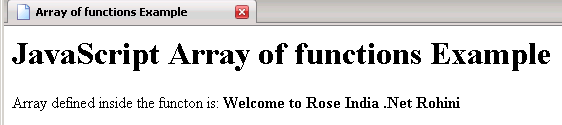Tutorials

# JavaScript array of functions

In this Tutorial we want to describe you a code that help in understanding a JavaScript array of functions. For this we are using Java Script language.

In this Tutorial we want to describe you a code that help in understanding a JavaScript array of functions. For this we are using Java Script language.

# JavaScript array of functions

In this Tutorial we want to describe you a code that help in understanding a JavaScript array of functions. For this we are using Java Script language. We declare a function  variable x that is used to store the build array( ) function specify an array1 variable that is used to hold the array object  indicate the size of element. The function show an array declare a variable hold the string. The for loop execute and run the script till variable i is less the array length. Finally the document. write print the function show Array (x ) that is used to hold the string variable and  print the x variable that hold the build function  passed as argument in function show array( ).

Javascript_Array_of_functions.html

 ```

JavaScript Array of functions Example

Array of functions Example                  ```

Output of the program